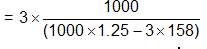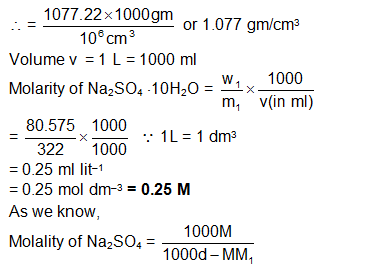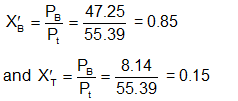×#### Thank you for registering.

One of our academic counsellors will contact you within 1 working day.

Click to Chat

1800-1023-196

+91-120-4616500

CART 0

• 0

MY CART (5)

Use Coupon: CART20 and get 20% off on all online Study Material

ITEM
DETAILS
MRP
DISCOUNT
FINAL PRICE
Total Price: Rs.

There are no items in this cart.
Continue Shopping• Complete JEE Main/Advanced Course and Test Series
• OFFERED PRICE: Rs. 15,900
• View Details

```Solved Examples on Solutions

Question: 1.The density of a 3M sodium thiosulphate solution is 1.25 gm cm–3. Calculate

i)   the molalities of Na+ and S2O32–  ions

ii)   percentage of weight of solution

iii)  mol-fraction of sodium thiosulphate.

Solution:

3 M Na2S2O3 (Sodium thiosulphate) solution means

3 moles Na2S2O3 is present in 1 L or, 1000 ml solution

Wt. of solute Na2S2O3 = 3×158

wt. of solution = v × d

= 1000 ml × 1.25 gm/ml

= 1000 × 1.25 gm

Wt. of solvent = (1000 × 1.25 – 3 ×158) gm H2O

Molality = no. of moles of solute per 1000 gm solvent= 3.865 mol kg–1 solvent

Now, Na2S2O3  2Na+ +S2O3-

a) Hence molality of Na+ = 2 ×3.865 mol kg-1

= 7.73 mol kg–1

Hence molality  = 1× 3.865 mol kg–1

= 3.865 mole/kg

b) % of wt. of solution

1 L i.e. 1000 ml solution containing 3 moles Na2S2O3

1000 × 1.25 gm solution containing 3 ×158 gm Na2S2O3Question:2. 8.0575 ×  10–2  kg of glauber’s salt (Na2SO4.10H2O) is dissolved in water to obtain 1 dm3 of a solution of density 1077.2 kgm–3. Calculate the molality, molarity and mole fraction of Na2SO4 in the solution.

Solution:

Wt. of Glauber’s satt = w1 = 8.0575 × 10–2 kg

= 80.575 gm

density of solution = d = 1077.2 kg m–3Question:3. A liquid mixture of benzene (mole fraction 0.33) and toluene (mole fraction 0.67) is taken. What will be the composition of vapours over the liquid mixture? This vapours is collected, condensed to a liquid and then allowed to evaporate so as to come into equilibrium with its vapour. What is composition of new vapour [Temperature = 20°C]. Given is vapour pressure of pure benzene = 75 torr at 20°C and vapour pressure of pure toluene = 22 torr)

Solution: Pt = 75 ×0.33 + 22 0.67 = 24.75 + 14.74 = 39.49 torr

The composition of t
he vapour in mole fraction units can be obtained by using Dalton’s law. In vapour phase we have;Again

Now the mole fraction of benzene and toluene in liquid are 0.63 and 0.37 respectively. Therefore, their vapour pressures are given by

Pt = 0.63 × 75 + 0.37 × 22 = 47.25 + 8.14 = 55.39 torr

Now we can calculate the mole fractions in vapour phase to be```### Course Features

• 728 Video Lectures
• Revision Notes
• Previous Year Papers
• Mind Map
• Study Planner
• NCERT Solutions
• Discussion Forum
• Test paper with Video Solution